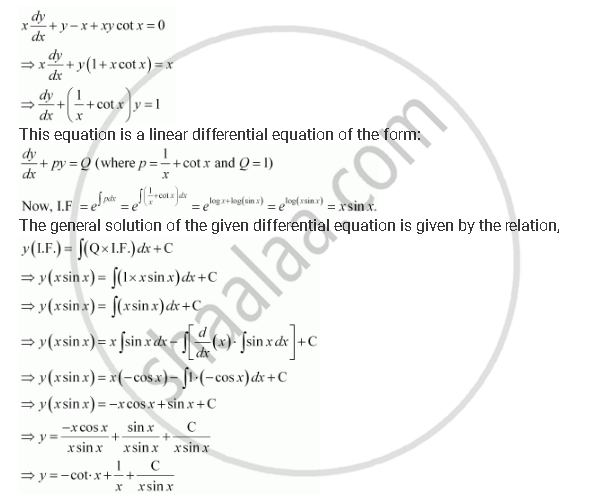Share

# For the Differential Equations Find the General Solution: X Dy/Dx + Y - X + Xycot X = 0(X != 0) - CBSE (Commerce) Class 12 - Mathematics

ConceptMethods of Solving First Order, First Degree Differential Equations Linear Differential Equations

#### Question

For the differential equations find the general solution:

x dy/dx + y - x + xycot x = 0(x != 0)

#### SolutionIs there an error in this question or solution?

#### APPEARS IN

NCERT Solution for Mathematics Textbook for Class 12 (2018 to Current)
Chapter 9: Differential Equations
Q: 9 | Page no. 414

#### Video TutorialsVIEW ALL 

Solution For the Differential Equations Find the General Solution: X Dy/Dx + Y - X + Xycot X = 0(X != 0) Concept: Methods of Solving First Order, First Degree Differential Equations - Linear Differential Equations.
S